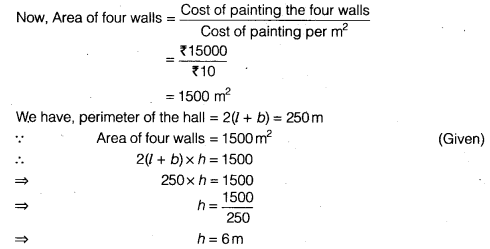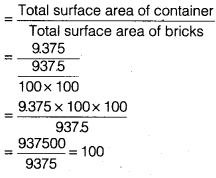NCERT Solutions for Class 9 Maths Chapter 13 Surface Areas and Volumes Ex 13.1 are part of NCERT Solutions for Class 9 Maths. Here we have given NCERT Solutions for Class 9 Maths Chapter 13 Surface Areas and Volumes Ex 13.1.

 Board CBSE Textbook NCERT Class Class 9 Subject Maths Chapter Chapter 13 Chapter Name Surface Areas and Volumes Exercise Ex 13.1 Number of Questions Solved 8 Category NCERT Solutions

## NCERT Solutions for Class 9 Maths Chapter 13 Surface Areas and Volumes Ex 13.1

Question 1.
A plastic box 1.5 m long, 1.25 m wide and 65 cm deep is to be made. It is opened at the top. Ignoring the thickness of the plastic sheet, determine
(i) The area of the sheet required for making the box.
(ii) The cost of sheet for it, if a sheet measuring 1m2 costs ₹20.
Solution:
We have a plastic box of
l = length = 15 m
b = width = 1.25 m
h = depth = 65 cm
= $$\frac { 65 }{ 100 }$$ m= 0.65 m (∵ 1 m = 100cm)
Surface area of the box = 2 (lb + bh + hl)
= 2(1.5 x 1.25 + 1.25 x 0.65 + 0.65 x 1.5)
= 2(1.875 + 0.8125 + 0.975) = 2 (3.6625)
= 7.325 m2
(i) Area of the sheet required for making the box
= 7.325 – l x b (∵ BOX is opened at the top)
= 7.325 – 1.5 x 1.25
= 7.325 – 1.875 = 5.45 m2
(ii) A sheet measuring 1 m2 costs = ₹20
∴ Sheet measuring 5.45 m2 costs = ₹20 x 5.45 = ₹109

Question 2.
The length, breadth and height of a room are 5 m, 4 m and 3 m, respectively. Find the cost of white washing the walls of the room and the ceiling at the rate of ₹17.50 per m2.
Solution:
We have a room of l = 5m
b = 4m
h = 3m
Required area for white washing
= Area of the four walls + Area of ceiling
= 2(l+ b) x h+ (l x b)
= 2(5+4) x 3 +(5 x 4)
= 2x 9x 3 + 20
= 54+20
= 74 m2
White washing 1 m2 costs = ₹7.50
White washing 74 m2 costs = ₹7.50x 74 = ₹555

Question 3.
The floor of a rectangular hall has a perimeter 250 m. If the cost of painting the four walls at the rate of ₹10 per m2 is ₹15000, find the height of the hall.
[Hint Area of the four walls = Lateral surface area]
Solution:
Let the rectangular hall of length = l, breadth = b, height = hHence, the height of the hall is 6 m.

Question 4.
The paint in a certain container is sufficient to paint an area equal to 9.375 m2. How many bricks of dimensions 22.5 cm x 10 cm x 7.5 cm can be painted out of this container.
Solution:
Given, dimensions of a brick
l = 22.5 cm, b = 10 cm
and b = 7.5cm
Total surface area of bricks = 2 ( l x b + b x h + h x l)
= 2(22.5 x 10 + 10 x 75 + 75 x 225)
= 2(225 + 75 + 168.75)
= 2 x 468.75 cm2
= 9375 cm2Number of bricks that painted out of this containerQuestion 5.
A cubical box has each edge 10 cm and another cuboidal box is 12.5 cm long, 10 cm wide and 8 cm high.
(i) Which box has the greater lateral surface area and by how much?
(ii) Which box has the smaller total surface area and by how much?
Solution:
We have l1 for cubical box = 10 cm
For cuboidal box l= 12.5 cm
b = 10 cm
h = 8 cm
(i) Lateral surface area of cubical box = 4l2 = 4(10)2
= 4 x 100
= 400 cm2
Lateral surface area of cuboidal box = 4 (l + b) x h
= 2 (125 + 10) x 8
= 2 (225) x 8
= 45 x 8 = 360 cm2
(∵ Lateral surface area of cuboidal box) > (Lateral surface area of cuboidal box) (∵ 400 >360)
∴ Required area = (400 – 360) cm2 = 40 cm2
(ii) Total surface area of cubical box = 6l2 = 6(10)2 = 6x 100= 600 cm2
Total surface area of cuboidal box = 2 ( l x b + b x h + h x l)
= 2(125 x 10 + 10 x 8 + 8 x 125)
= 2(125 + 80+ 100)
= 2 x 305
= 610 cm2
∴ (Area of cuboidal box) > (Area of cubical box) (∵ 610 > 600)
Required area = (610 – 600)cm2 = 10 cm2

Question 6.
A small indoor greenhouse (herbarium) is made entirely of glass panes (including base) held together with tape. It is 30 cm long, 25 cm wide and 25 cm high.
(i) What is the area of the glass?
(ii) How much of tape is needed for all the 12 edges?
Solution:
Dimension for herbarium are
l = 30 cm, b = 25 cm and h = 25 cm
Area of the glass = 2 (l x b + b x h + h x l)
= 2 ( 30 x 25 + 25 x 25 + 25 x 30)
= 2(750 + 625 + 750) = 2 (2125) = 4250cm2
∴ Length of the tape = 4 (l + b + h) = 4(30 + 25 + 25)
[∵ Herbarium is a shape of cuboid length = 4 (1+ b + h)] = 4×80= 320cm

Question 7.
Shanti Sweets Stalll was placing an order for making cardboard boxes for packing their sweets. Two sizes of boxes were required. The bigger of dimensions 25 cm x 20 cm x 5 cm and the smaller of dimensions 15 cm x 12 cm x 5 cm. For all the overlaps, 5% of the total surface area is required extra. If the cost of the cardboard is ₹4 for 1000 cm2, find the cost of cardboard required for supplying 250 boxes of each kind.
Solution:
Dimension for bigger box, l = 25 cm, b = 20 cm and b = 5 cm
Total surface area of the bigger size box
=2 ( l x b + b x h + h x l)
= 2(25 x 20 + 20 x 5 + 5 x 25)
= 2(500+ 100+ 125)
= 2(725)= 1450 cm2
Dimension for smaller box, l = 15 cm b = 12 cm and h = 5 cm
Total surface area of the smaller size box = 2(15 x 12 + 12 x 5 + 5 x 15)
= 2 (180 + 60 + 75)= 2 (315)= 630 cm2
Area for all the overlaps = 5% x 2080 cm2 = $$\frac { 5 }{ 100 }$$ – x 2080 cm2 = 104 cm2
Total surface area of both boxes and area of overlaps = (2080 + 104) cm2 = 2184 cm2
Total surface area for 250 boxes = 2184 x 250 cm2
Cost of the cardboard for 1000 cm2 = ₹4
Costs of the cardboard for 1 cm = ₹ $$\frac { 4 }{ 1000 }$$
Cost of the cardboard for 2184 x 250 cm2 = ₹ $$\frac { 4x 2184 x 250 }{ 1000 }$$ = ₹ 2184

Question 8.
Parveen wanted to make a temporary shelter, for her car, by making a box-like structure with tarpaulin that covers all the four sides and the top of the car (with the front face as a flap which can be rolled up). Assuming that the stitching margins are very small and therefore negligible, how much tarpaulin would be required to make the shelter of height 2.5 m, with base dimensions 4 m x 3 m?
Solution:
Dimension for shetter, l = 4 m, b = 3 m and h = 25 cm
Required area of tarpaulin to make the shelter
= (Area of 4 sides + Area of the top) of the car
= 2(l + b) x h+ ( l x b)
= 2(4+ 3) x 25 + (4 x 3)
= (2 x 7 x 25) + 12 = 35 + 12
= 47 m2

We hope the NCERT Solutions for Class 9 Maths Chapter 13 Surface Areas and Volumes Ex 13.1 help you. If you have any query regarding NCERT Solutions for Class 9 Maths Chapter 13 Surface Areas and Volumes Ex 13.1, drop a comment below and we will get back to you at the earliest.